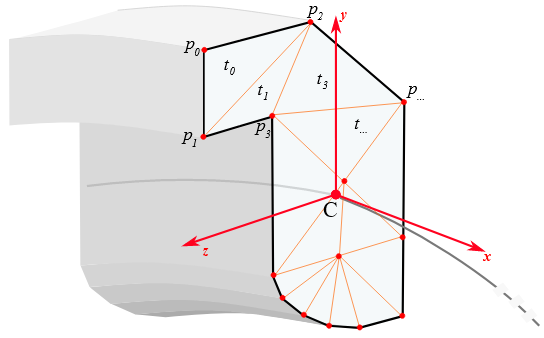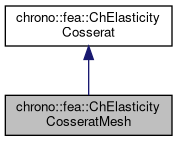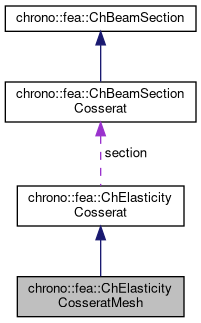chrono::fea::ChElasticityCosseratMesh Class Reference

## Description

Elasticity for a beam section in 3D, where the section is defined by a mesh of triangles.

This model saves you from the need of knowing I_z, I_y, A, etc., because the generalized n and m are automatically computed by integrating stresses on the triangulated section. Note that stresses are linearly interpolated between the vertexes of the triangle sections. Triangles can share vertexes. Each vertex has its own material. Section is assumed always flat, even if the section mesh is not connected, ex. if one models a section like a "8" shape where the two "o" are not connected.

Benefits:

• no need to provide I_y, I_z, A, etc.
• possibility of getting values of stresses in different points of the section
• possibility of using some 1D plasticity to discover plasticizing zones in the section

Limitations (TO BE REMOVED IN FUTURE using Vlasov / Prandtl theories):

• section torsional warping not included,
• torsion stresses are correct only in tube-like shapes, or similar; other models such as ChElasticityCosseratAdvanced contain torsional effects via the macroscopic J constant; here there is a torsion correction factor just for correcting the m_x result, but at this point, shear in material points would have less meaning.
• shear stresses (ex. cantilever with transverse load) should be almost parabolic in the section in reality, here would be constant. Other models such as ChElasticityCosseratAdvanced correct this effect at the macroscopic level using the Timoshenko correction factors Ks_y and Ks_z, here they are used as well, but if so, shear in material points would have less meaning.

This material can be shared between multiple beams.#include <ChBeamSectionCosserat.h>

Inheritance diagram for chrono::fea::ChElasticityCosseratMesh:[legend]
Collaboration diagram for chrono::fea::ChElasticityCosseratMesh:[legend]

## Public Member Functions

virtual std::vector< ChVector2<> > & Vertexes ()
Access the list of vertexes, to get/change/add mesh section vertexes.

std::vector< std::shared_ptr< ChSectionMaterial > > & Materials ()
Access the list of material(s), to get/change/add mesh section materials. More...

std::vector< ChVector< int > > & Triangles ()
Access the list of triangles, to get/change/add mesh section triangles. More...

virtual void SetAsRectangularSection (double width_y, double width_z)
Set rectangular centered section, using two triangles. More...

virtual void SetAsCircularSection (double diameter)
Set circular centered, using n triangles. More...

virtual void ComputeStress (ChVector<> &stress_n, ChVector<> &stress_m, const ChVector<> &strain_e, const ChVector<> &strain_k) override
Compute the generalized cut force and cut torque. More...Public Member Functions inherited from chrono::fea::ChElasticityCosserat
virtual void ComputeStiffnessMatrix (ChMatrixNM< double, 6, 6 > &K, const ChVector<> &strain_e, const ChVector<> &strain_k)
Compute the 6x6 tangent material stiffness matrix [Km] = dσ/dε, given actual deformation and curvature (if needed). More...

## Protected Attributes

std::vector< ChVector2<> > vertexes

std::vector< std::shared_ptr< ChSectionMaterial > > materials

std::vector< ChVector< int > > trianglesPublic Attributes inherited from chrono::fea::ChElasticityCosserat
ChBeamSectionCosseratsection

## ◆ ComputeStress()

 void chrono::fea::ChElasticityCosseratMesh::ComputeStress ( ChVector<> & stress_n, ChVector<> & stress_m, const ChVector<> & strain_e, const ChVector<> & strain_k )
overridevirtual

Compute the generalized cut force and cut torque.

Parameters
 stress_n local stress (generalized force), x component = traction along beam stress_m local stress (generalized torque), x component = torsion torque along beam strain_e local strain (deformation part): x= elongation, y and z are shear strain_k local strain (curvature part), x= torsion, y and z are line curvatures

Implements chrono::fea::ChElasticityCosserat.

## ◆ Materials()

 std::vector >& chrono::fea::ChElasticityCosseratMesh::Materials ( )
inline

Access the list of material(s), to get/change/add mesh section materials.

Each material correspond to an equivalent vertex. If there is only one material, it will be used for all vertexes.

## ◆ SetAsCircularSection()

 void chrono::fea::ChElasticityCosseratMesh::SetAsCircularSection ( double diameter )
virtual

Set circular centered, using n triangles.

Note: for testing only, use ChElasticityCosseratSimple instead. No material defined: you still must set E and G.

## ◆ SetAsRectangularSection()

 void chrono::fea::ChElasticityCosseratMesh::SetAsRectangularSection ( double width_y, double width_z )
virtual

Set rectangular centered section, using two triangles.

Note: for testing only, use ChElasticityCosseratSimple instead. No material defined: you still must set E and G.

## ◆ Triangles()

 std::vector >& chrono::fea::ChElasticityCosseratMesh::Triangles ( )
inline

Access the list of triangles, to get/change/add mesh section triangles.

Each triangle has three integer indexes pointing to the three connected vertexes in the Vertexes() array, where 0 is the 1st vertex etc.

The documentation for this class was generated from the following files:
• /builds/uwsbel/chrono/src/chrono/fea/ChBeamSectionCosserat.h
• /builds/uwsbel/chrono/src/chrono/fea/ChBeamSectionCosserat.cpp